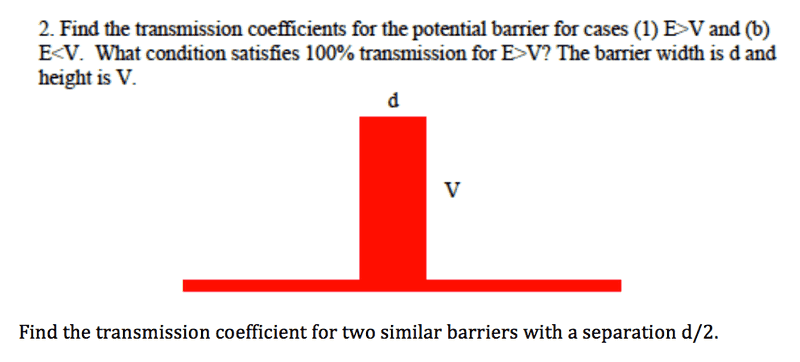# Transmission Coefficient for Quantum Barrier

• BenBa

## Homework Statement

Picture of Problem:## Homework Equations

$$\psi(x) = A_n e^{ikx} + B_n e^{-ikx}$$ for n=1,2,3

## The Attempt at a Solution

I know i need to relate the wave functions $$A_n e^{ikx} + B_n e^{-ikx}$$ for n=1,2,3 (the three areas of the barrier - before barrier, inside barrier, after barrier), such that the values of the functions at the barrier edges as well as their derivatives are equal. But i am not sure how to solve for each coefficient. Also how does the length of the barrier, d, come into play?

k is equal to $$\sqrt{\frac{2mE}{\hbar^2}}$$ outside the barrier and $$\sqrt{\frac{2m(E-V)}{\hbar^2}}$$ inside the barier.

Last edited:

I know i need to relate the wave functions $$A_n e^{ikx} + B_n e^{-ikx}$$ for n=1,2,3 (the three areas of the barrier - before barrier, inside barrier, after barrier), such that the values of the functions at the barrier edges as well as their derivatives are equal.